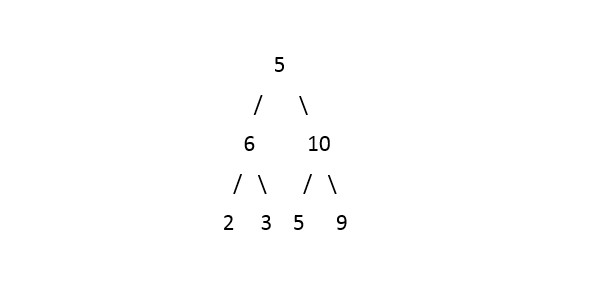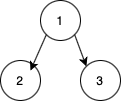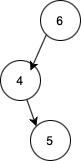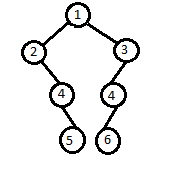Problem title
Difficulty
Avg time to solve

Rearrange The Array
Moderate
15 mins
Trailing Zeros in Factorial
Moderate
15 mins
Dijkstra's shortest path
Moderate
25 mins
Construct Binary Tree From Inorder and Preorder Traversal
Easy
10 mins
Check If Binary Representation of a Number is Palindrome
Easy
15 mins
Maximum of All Subarrays of Size K
Easy
15 mins
Common Elements In Three Sorted Arrays
Moderate
35 mins
Minimize Cash Flow
Easy
15 mins
Three Way Partition
Moderate
15 mins
Longest Common Substring
Moderate
25 mins18

# Largest Number in Binary Tree

Difficulty: MEDIUM
Avg. time to solve
36 min
Success Rate
60%

Problem Statement

#### Your task is to find the largest number that could be formed by concatenating all its nodes values.

##### For example:
``````For the given binary tree:
````````````Output: 96553210

Explanation: After concatenating all the numbers in the above binary tree this is the largest number that can be formed.
``````
##### Input Format:
``````The first line of input contains a single integer 'T', representing the number of test cases.

The only line of each test case contains elements in the level order form. The line consists of values of nodes separated by a single space. In case a node is null, we take -1 in its place.

Example:
The input for the tree depicted in the below image would be:
1 2 3 4 -1 5 6 -1 7 -1 -1 -1 -1 -1 -1
``````#### Explanation :

``````Level 1 :
The root node of the tree is 1

Level 2 :
Left child of 1 = 2
Right child of 1 = 3

Level 3 :
Left child of 2 = 4
Right child of 2 = null (-1)
Left child of 3 = 5
Right child of 3 = 6

Level 4 :
Left child of 4 = null (-1)
Right child of 4 = 7
Left child of 5 = null (-1)
Right child of 5 = null (-1)
Left child of 6 = null (-1)
Right child of 6 = null (-1)

Level 5 :
Left child of 7 = null (-1)
Right child of 7 = null (-1)

1
2 3
4 -1 5 6
-1 7 -1 -1 -1 -1
-1 -1
``````
##### Note:
``````The first node that is not null (of the previous level) is treated as the parent of the first two nodes of the current level. The second not-null node (of the previous level) is treated as the parent node for the next two nodes of the current level and so on. The input ends when all nodes at the last level are null (-1).
``````
##### Output Format:
``````For each test case, print the integer that represents the largest number that could be formed by concatenating all its nodes given in a Binary Tree.
``````
##### Note:
``````You don't need to print anything, it has already been taken care of. Just implement the given function.
``````
##### Constraints:
``````1 <= T <= 5
0 <= N <= 10^4
0 <= data <= 10^3

Where 'N' denotes the total number of nodes and 'data' denotes the node value of the Binary Tree.

Time limit: 1 sec
``````
##### Sample Input 1:
``````2
1 2 3 -1 -1 -1 -1
6 4 -1 -1 5 -1 -1
``````
##### Sample Output 1:
``````321
654
``````
##### Explanation to Sample Output 1:
``````In test case 1, the input Binary Tree will be represented as:
````````````From all possible permutations of concatenated integers in the above Binary Tree, the largest number possible is 321.

In test case 2, the input Binary Tree will be:
````````````From all possible permutations of concatenated integers in the above Binary Tree, the largest number possible is 654.
``````
##### Sample Input 2:
``````2
1 2 3 -1 4 4 -1 -1 5 6 -1 -1 -1 -1 -1
1 2 3 4 -1 5 6 -1 7 -1 -1 -1 -1 -1 -1
``````
##### Sample Output 2:
``````6544321
7654321
``````
##### Explanation to Sample Output 2:
``````In test case 1, the input Binary Tree will be represented as:
````````````From all possible permutations of concatenated integers in the above Binary Tree, the largest number possible is 6544321.

In test case 2, the input Binary Tree will be represented as shown in the Input Format section above. From all possible permutations of concatenated integers in the above Binary Tree, the largest number possible is 7654321.
``````Console